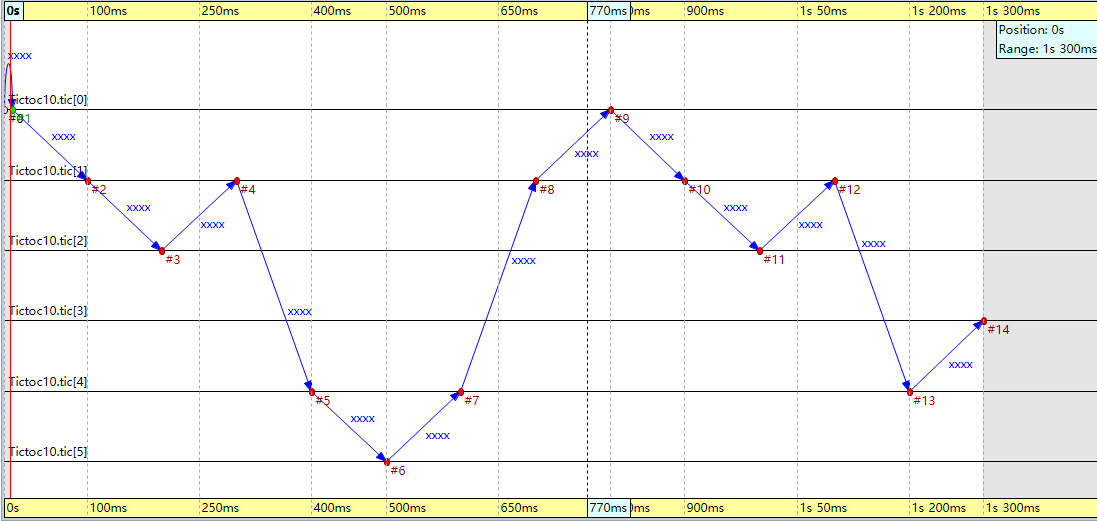# omnet++ 示例13练习题

Exercise: you’ll notice that this simple “routing” is not very efficient: often the packet keeps bouncing between two nodes for a while before it is sent to a different direction. This can be improved somewhat if nodes don’t send the packet back to the sender. Implement this. Hints: cMessage::getArrivalGate(), cGate::getIndex(). Note that if the message didn’t arrive via a gate but was a self-message, then getArrivalGate() returns NULL. 。

1. 会出现getSize=1的情况，即消息所在的节点只有一个连接节点，那么此时消息只能原路返回
2. 示例中提到的self-message情况，此时的arrivalgate=NULL

forwardMessage函数的代码如下：

void Txc10::forwardMessage(cMessage *msg)
{
int n = gateSize("out");
EV << "The number of gates:" << n << "\n";
int k = intuniform(0, n-1);
cGate *arrivalGate = msg->getArrivalGate();
if((arrivalGate == NULL)||(n==1))
{
EV << "Forwarding message " << msg << " on port out[" << k << "]\n";
send(msg, "out", k);
}
else{
int arrivalGateIndex = arrivalGate->getIndex();
EV << "arrivalGateIndex: " << arrivalGateIndex << ".\n";
while(k == arrivalGateIndex)
k = intuniform(0, n-1);
EV << "Forwarding message " << msg << " on port out[" << k << "]\n";
send(msg, "out", k);
}
}12-1028602-066246
12-25360
07-03166
09-213059
01-10
11-28
01-10
07-19
06-1817
02-192614
07-13
©️2020 CSDN 皮肤主题: 大白 设计师:CSDN官方博客